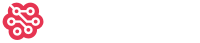## Questions counted by vote range

1

(click on this box to dismiss)Q&A for users and developers of hardware and software for Raspberry Pi

``````SELECT
COUNT(*) * 100.0 / SUM(COUNT(*)) OVER () AS PercentAllQuestions,
COUNT(CASE WHEN IsOpenEnded = 1 THEN 1 END) * 100.0 / SUM(COUNT(CASE WHEN IsOpenEnded = 1 THEN 1 END)) OVER () AS PercentOpenEndedQuestions,
ScoreCategory
FROM (
SELECT
CASE
WHEN Score < 0 THEN 'Negative'
WHEN Score = 0 THEN 'Zero'
WHEN Score BETWEEN 1 AND 5 THEN '1-5'
WHEN Score BETWEEN 6 AND 9 THEN '6-9'
WHEN Score BETWEEN 10 AND 20 THEN '10-20'
WHEN Score > 20 THEN '>20'
ELSE 'Bug'
END AS ScoreCategory,
CASE
WHEN Score < 0 THEN -1
WHEN Score = 0 THEN 0
WHEN Score BETWEEN 1 AND 5 THEN 1
WHEN Score BETWEEN 6 AND 9 THEN 6
WHEN Score BETWEEN 10 AND 20 THEN 10
WHEN Score > 20 THEN 20
ELSE 'Bug'
END AS ScoreCategoryNum,
CASE
WHEN UPPER(Posts.Tags) LIKE '%<OPEN-ENDED>%' THEN 1
ELSE 0
END AS IsOpenEnded
FROM Posts
JOIN PostTypes ON PostTypes.Id = Posts.PostTypeId
WHERE PostTypes.Name = 'Question'
) QuestionsByCategory
GROUP BY ScoreCategory, ScoreCategoryNum
ORDER BY ScoreCategoryNum``````

### Enter Parameters

Options:
-Hold tight while we fetch your results
:records returned in :time ms:cached